# 3 4 Scientific Notation Warm Up Problem of

• Slides: 183 -4 Scientific Notation Warm Up Problem of the Day Lesson Presentation Course 1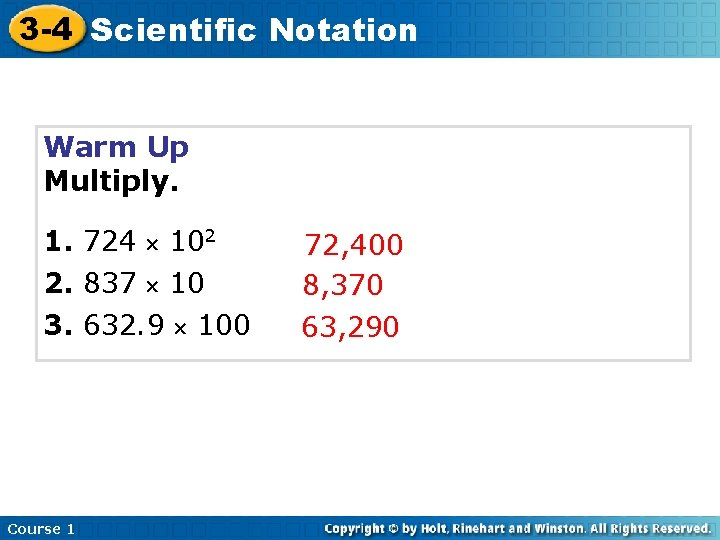3 -4 Scientific Notation Warm Up Multiply. 1. 724 102 2. 837 10 3. 632. 9 100 Course 1 72, 400 8, 370 63, 290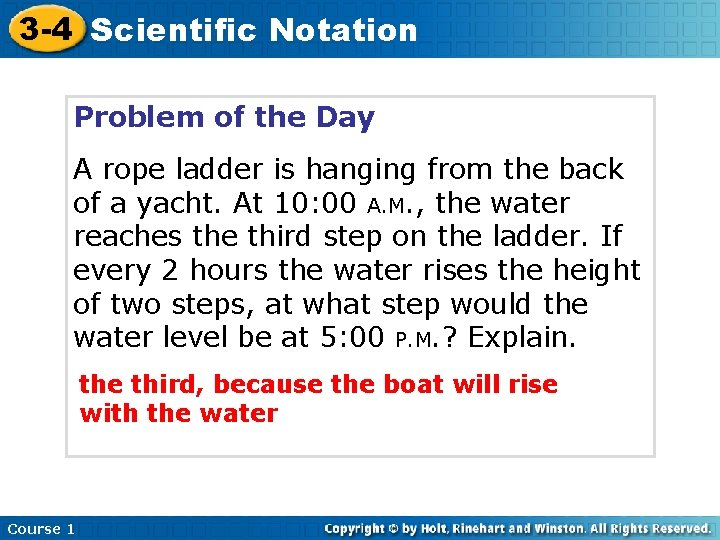3 -4 Scientific Notation Problem of the Day A rope ladder is hanging from the back of a yacht. At 10: 00 A. M. , the water reaches the third step on the ladder. If every 2 hours the water rises the height of two steps, at what step would the water level be at 5: 00 P. M. ? Explain. the third, because the boat will rise with the water Course 1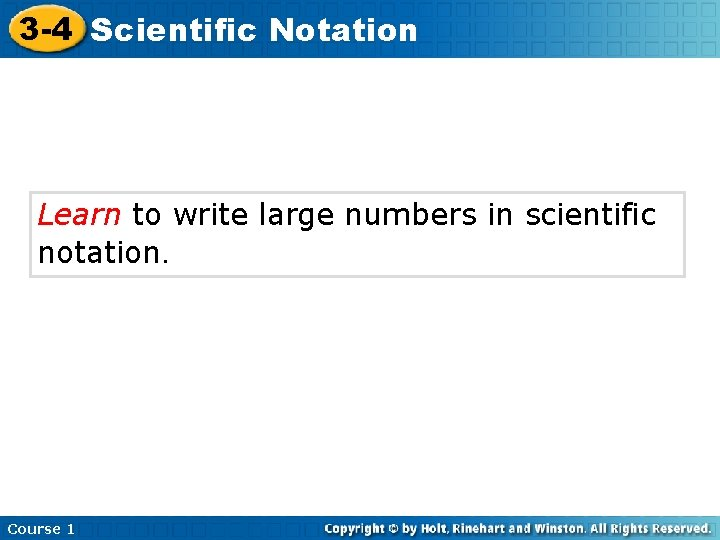3 -4 Scientific Notation Learn to write large numbers in scientific notation. Course 1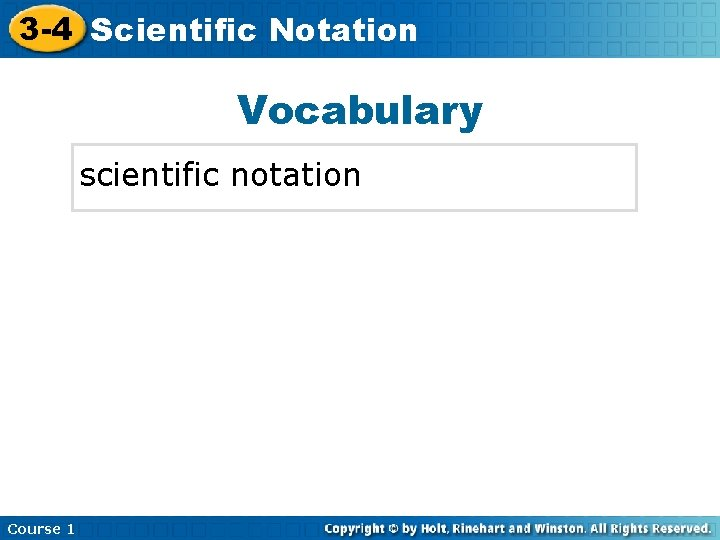3 -4 Scientific Insert Lesson Title Here Notation Vocabulary scientific notation Course 13 -4 Scientific Notation Additional Example 1 A: Multiplying by Powers of Ten Find each product. 5, 892 1, 000 5, 892. 000 There are 3 zeros in 1, 000. To multiply, move the decimal 3 places right. = 5, 892, 000 Course 1 Write 3 placeholder zeros.3 -4 Scientific Notation Additional Example 1 B: Multiplying by Powers of Ten Find each product. 47. 75 10, 000 47. 7500 There are 4 zeros in 10, 000. To multiply, move the decimal 4 places right. = 477, 500 Course 1 Write 4 placeholder zeros.3 -4 Scientific Notation Check It Out: Example 1 A Find each product. 901 1, 000 901. 000 There are 3 zeros in 1, 000. To multiply, move the decimal 3 places right. = 901, 000 Course 1 Write 3 placeholder zeros.3 -4 Scientific Notation Check It Out: Example 1 B Find each product. 15. 425 10, 000 15. 4250 There are 4 zeros in 10, 000. To multiply, move the decimal 4 places right. = 154, 250 Course 1 Write 4 placeholder zeros.3 -4 Scientific Notation Scientific notation is a shorthand method for writing large numbers. A number written in scientific notation has two numbers that are multiplied. 3. 456 106 The first part is a number that is greater than or equal to 1 and less than 10. Course 1 The second part is a power of 10.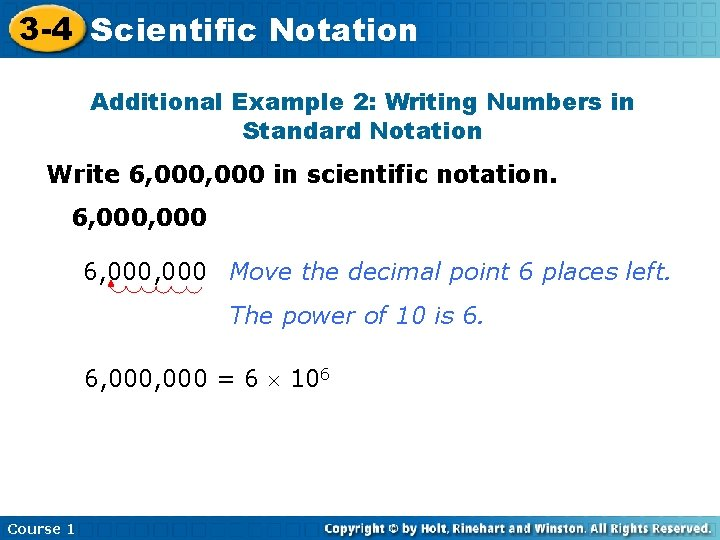3 -4 Scientific Notation Additional Example 2: Writing Numbers in Standard Notation Write 6, 000 in scientific notation. 6, 000, 000 Move the decimal point 6 places left. The power of 10 is 6. 6, 000 = 6 106 Course 1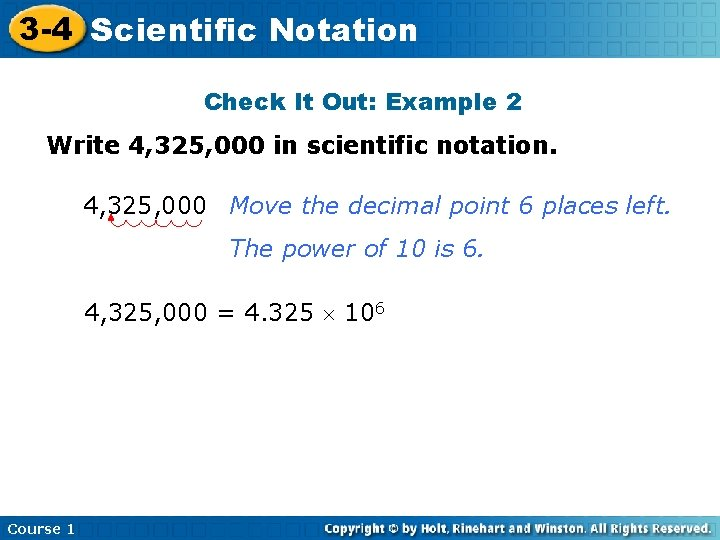3 -4 Scientific Notation Check It Out: Example 2 Write 4, 325, 000 in scientific notation. 4, 325, 000 Move the decimal point 6 places left. The power of 10 is 6. 4, 325, 000 = 4. 325 106 Course 13 -4 Scientific Notation You can write a large number written in scientific notation in standard form. Look at the power of 10 and move the decimal point that number of places to the right. Course 1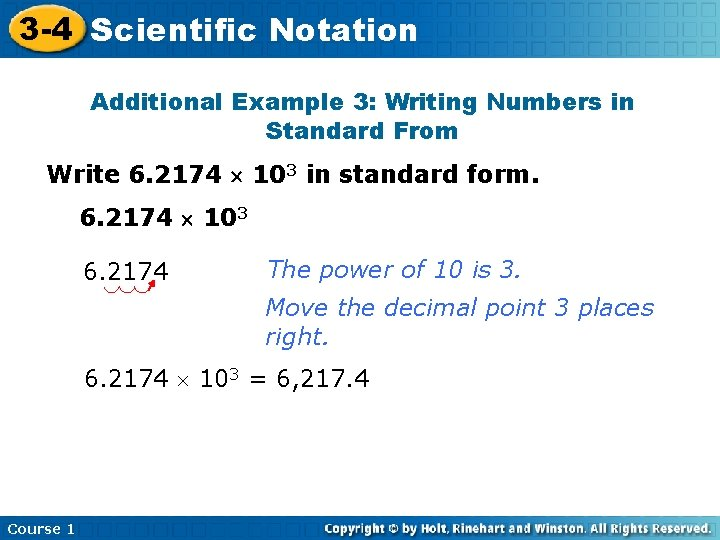3 -4 Scientific Notation Additional Example 3: Writing Numbers in Standard From Write 6. 2174 103 in standard form. 6. 2174 103 6. 2174 The power of 10 is 3. Move the decimal point 3 places right. 6. 2174 103 = 6, 217. 4 Course 13 -4 Scientific Notation Check It Out: Example 3 Write 3. 21975 103 in standard form. 3. 21975 103 3. 21975 The power of 10 is 3. Move the decimal point 3 places right. 3. 21975 103 = 3, 219. 75 Course 1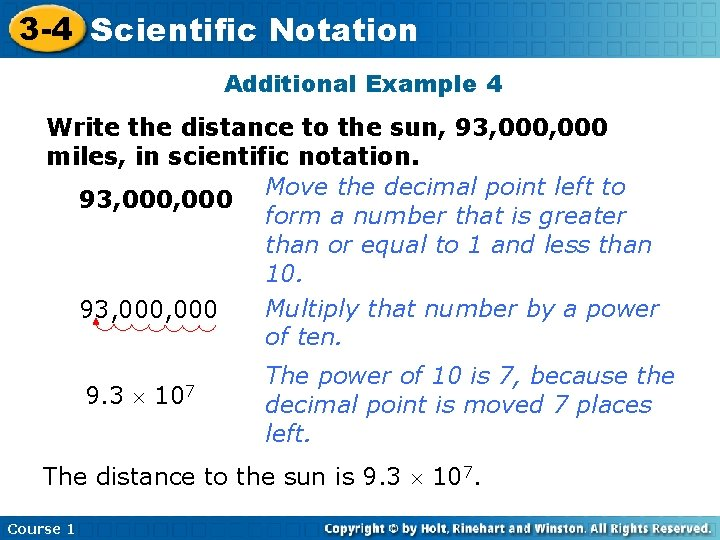3 -4 Scientific Notation Additional Example 4 Write the distance to the sun, 93, 000 miles, in scientific notation. Move the decimal point left to 93, 000 form a number that is greater than or equal to 1 and less than 10. Multiply that number by a power 93, 000 of ten. 9. 3 107 The power of 10 is 7, because the decimal point is moved 7 places left. The distance to the sun is 9. 3 107. Course 1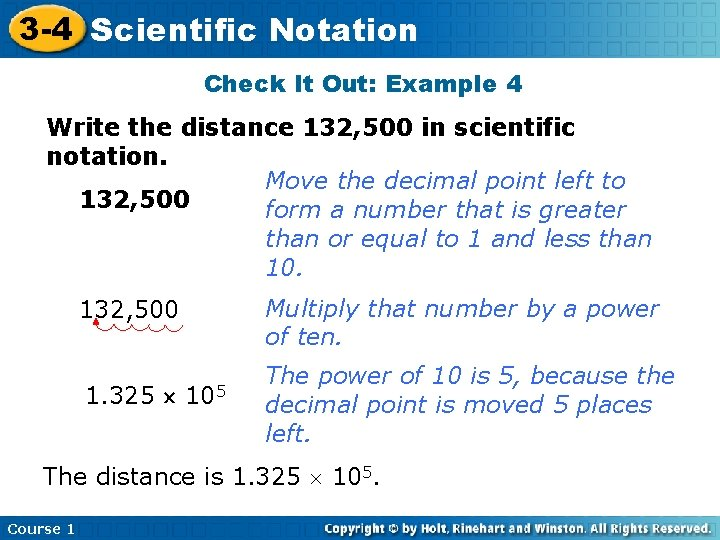3 -4 Scientific Notation Check It Out: Example 4 Write the distance 132, 500 in scientific notation. Move the decimal point left to 132, 500 form a number that is greater than or equal to 1 and less than 10. 132, 500 1. 325 105 Multiply that number by a power of ten. The power of 10 is 5, because the decimal point is moved 5 places left. The distance is 1. 325 105. Course 1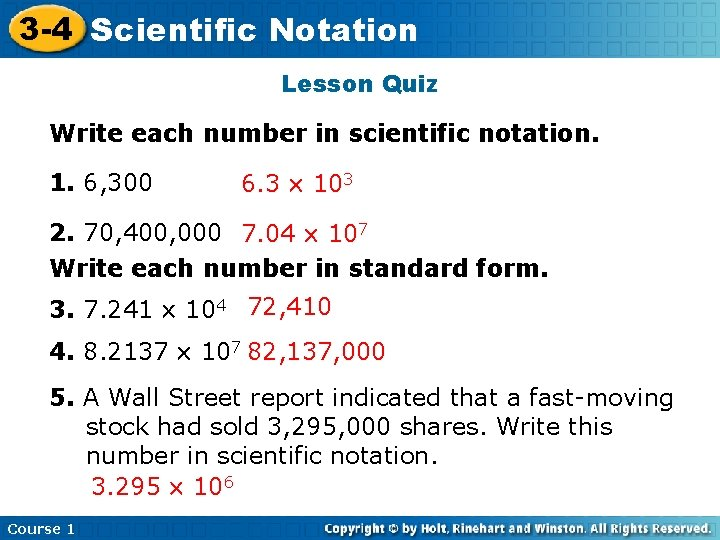3 -4 Scientific Insert Lesson Notation Title Here Lesson Quiz Write each number in scientific notation. 1. 6, 300 6. 3 103 2. 70, 400, 000 7. 04 107 Write each number in standard form. 3. 7. 241 104 72, 410 4. 8. 2137 107 82, 137, 000 5. A Wall Street report indicated that a fast-moving stock had sold 3, 295, 000 shares. Write this number in scientific notation. 3. 295 106 Course 1